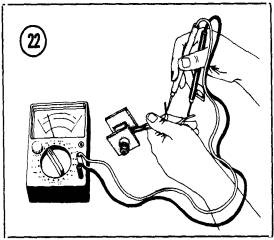RECTIFIER

The rectifier assembly serves two purposes. It converts alternating current produced by the alternator into direct current, which is used to charge the battery. It also prevents discharge of the battery through the alternator when the engine isn’t running, or at other times when the output voltage of the alternator is less than bat­tery voltage.

KH250, KH400, and S Series Rectifier Troubleshooting1.  Measure resistance between each yellow lead and the red lead (Figure 22). Record each ohm­meter reading.

2.  Reverse the ohmmeter leads and repeat Step 1.

3.  If each pair of measurements was essentially infinite in one direction and low in the reverse direction, proceed with Step 4. If any pair of measurements was either high or low in both directions, replace the rectifier assembly.

5.  Reverse the meter connections and repeat Step 4. If any pair of measurements was either high or low in both directions, replace the rectifier assembly.

KH500 and H1 Rectifier Troubleshooting

The HI rectifier is similar to that for S series models, except that it has one additional blue lead to be checked. Proceed as follows:

1.  Measure resistance between each yellow wire to the red wire. Record the meter indications.

2.  Reverse the meter leads and repeat the measurements.

3.  If each pair of measurements was essentially infinite in one direction and low in the reverse direction, proceed with Step 4. If any pair of measurements was either high or low in both directions, replace the rectifier.

4.  Measure the resistance between each yellow wire and the black wire. Record the meter in­dications.

5.  Reverse the meter leads and repeat the measurements. If any pair of measurements was either high or low in both directions, re­place the rectifier. If OK, proceed with Step 6.

6.  Measure resistance between the black and blue wires, then reverse the meter leads and repeat the measurement. If the meter indicates low resistance in one direction and high re­sistance with the leads reversed, the rectifier is OK. If both measurements are either high or low, replace the rectifier.

H2 Voltage Regulator/Rectifier Troubleshooting

Note: Also see http://kawatriple.com/bulletins/73-h9.pdf

 RegulatorThe H2 rectifier unit performs the dual functions of current rectification and voltage regu­lation. To check the unit, proceed as follows:

 Figure 23

1. Refer to Figure 23.  Measure resistance be­tween the black and red leads, then repeat the measurement with the ohmmeter leads re­versed. The meter should indicate approximately 70 ohms in one direction and 1,000 ohms in the other. If OK, proceed to Step 2

2.  Measure resistance between the black lead and each yellow lead, then repeat the measure­ments with the meter leads reversed. Resistance should be approximately 25 ohms in one direc­tion and 1,000 ohms in the other.

3.  Measure resistance between each yellow lead and the red lead, then reverse the meter leads and repeat the measurements. Both sets of readings should be approximately 25 ohms in one direction. In the reverse direction, one reading should be approximately 1,000 ohms and the other should be approximately 4,000 ohms.4. Connect the circuit shown in Figure 24 using a suitable power supply. Then measure re­sistance between the two yellow leads. Resistance should be essentially infinite in one direction and approximately 500 ohms with the ohmmeter leads reversed.

5. Reverse the power supply polarity, and lower its output voltage, as shown in Figure 25. Measure resistance between both yellow leads.  Resistance should be essentially infinite in both directions.

6.  Replace the rectifier unit if it fails any of the foregoing tests.